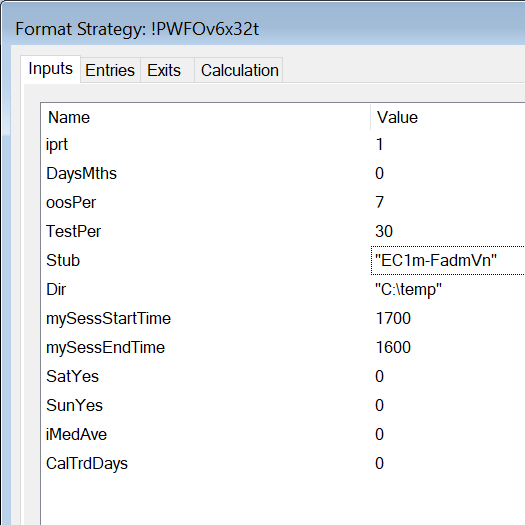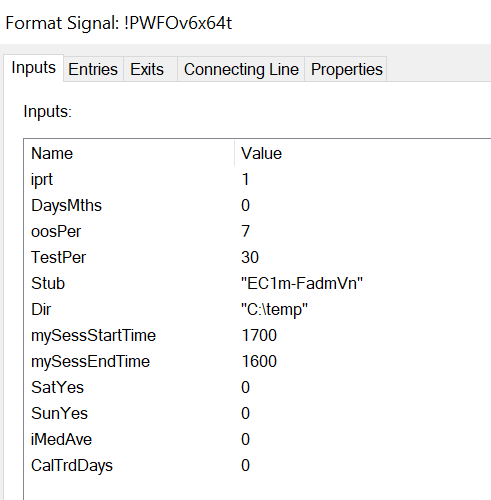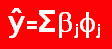Order Online Power Walk Forward
Optimizer
Walk Forward
Metric Explorer
Walk Forward
Input Explorer
Walk Forward
Surface Explorer
Key Daily & Intraday
Nth Order Fixed Memory
Polynomial Strategy
Nth Order Fading Memory
Polynomial Strategy
End Point Fast Fourier
Transform Strategy
Goertzel DFT
Strategy
Five Parameter
Parabolic Strategy
Dennis Meyers
Working Papers

# Power Walk Forward Optimizer Strategy Form Description## PWFO v6 Strategy Input Definitions

iprt(0)
iprt=1 means the dll will calculate the PWFO performance metrics shown in the PWFO description and print the results to the files named in FileID. iprt=0 means that no output will be printed and the dll will not be used by the strategy.
DaysMths(0
DaysMths is a switch that indicates whether oosPer and TestPer are in calendar days, trading days or monthly periods. If DaysMths=0 then oosPer and TestPer are periods calculated in calendar days (CalTrdDays=0), or trading days (CalTrdDays=1). If DaysMths=1 then oosPer and TestPer are monthly periods. For instance, if DaysMths=1 and oosPer=3 and TestPer=12 then the PWFO test period is 12 calendar months and the out-of-sample period is 3 calendar months. Note: calendar months follow exactly the monthly periods of January, February, March, April, May, June, July, August, September, October, November and December. There are no intra-month months.
oosPer (7)
This is the number of calendar days or trading days or monthly periods of out-of-sample performance that you wish to see on the printout line for each case, Note: When oosPer is set to zero then the PWFO will run only the latest TestPer days and will create a file with just the Test data performance results and no out-of-sample results. This was done so that you could use the PWFO to generate the output performance results for the latest TestPer days to choose the strategy input parameters to use for trading the upcoming period. If DaysMths=1 then oosPer is the number of exact calendar months.
TestPer(30)
This is the number of calendar days or trading days or monthly periods of the in-sample data that you wish to use for each case in your optimization run, If DaysMths=1 then TestPer is the number of exact calendar months.
Stub is part of the file name that the performance statistics will be written too. This can be any name that you choose, but I try to make the stub into "Trading symbol+Time Bars+Strategy". Thus, es1fadm would be 1-minute bars of the E-Mini Futures using the FADM strategy.. Note: Be sure to include the double quotation marks when naming a new stub for the Field input. The output file name is constructed as filename = Dir+Stub+LastOOSDay.csv . OOSDay is the last day of the out-of-sample section (see below).
Dir("C:\Temp")
Dir is the disk drive and directory that you wish the PWFO Excel files with the performance statistics to be written too. This can be any directory that you choose. As mentioned above the Excel output file name, that the PWFO statistics are written too, is constructed as filename=Dir+Stub+LastOOSDay.csv
mySessStartTime(0), mySessEndTime(2400)
This defines when the security or future, that you are using on a chart, session time starts and ends. . Here is an example of the use of MySessStartTime and mySessEndTime: EC is traded on CME Globex and its session start time is 1700 CME time and its session end time is 1600, so you would put mySessStartTime=1700 and mySessEndTime=1600. NOTE: if your future/stock is not a 24-hour future and only trades during the day hours, then put mySessStartTime=0 and mySessEndTime=2400.
SatYes(0)
Currently not used but reserved for future expansion
SunYes(0 or 1)
If CalTrdDays=1 then SunYes=1 includes Sunday in the trading days. If CalTrdDays=1 and SunYes=0 then only weekdays Monday thru Friday are included in trading days. If CalTrdDays=0 then SunYes is not used.
CalTrdDays(0 or 1)
If CalTrdDays=0 then the oosPer and TestPer are calculated using calendar days. If CalTrdDays=1 then the oosPer and TestPer are calculated using the trading days Monday thru Friday and Sunday(if SunYes=1. Sun not included if SunYes=0). As an example, if CalTrdDays=1, SunYes=0,and TestPer=5, the algorithm would count back 5 trading days excluding Sat and Sun in the count and use the date found 5 Trading days back.
iMedAve(0 or 1)
If iMedAve=0 then the median is used in the metrics that use the median. If iMedAve=1 then the average is used instead of the median in the metrics that used the median.Top | Home